# 3 First Look at Lists

 3 First Look at Lists
Lists are a basic way to collect things together in the Wolfram Language. {1,2,3} is a list of numbers. On their own, lists don’t do anything; they’re just a way to store things. So if you give a list as input, it’ll just come back unchanged:
{1, 2, 3, 4, a, b, c}

ListPlot is a function that makes a plot of a list of numbers.
Plot the list of numbers {1, 1, 2, 2, 3, 4, 4}:
ListPlot[{1, 1, 2, 2, 3, 4, 4}]

Plot the list of numbers {10, 9, 8, 7, 3, 2, 1}:
ListPlot[{10, 9, 8, 7, 3, 2, 1}]

Range is a function that makes a list of numbers.
Generate a list of numbers up to 10:
Range

ListPlot[Range]

Reverse reverses the elements in a list.
Reverse the elements in a list:
Reverse[{1, 2, 3, 4}]

Reverse what Range has generated:
Reverse[Range]

Plot the reversed list:
ListPlot[Reverse[Range]]

Join joins lists together, making a single list as the result.
Join lists together:
Join[{1, 2, 3}, {4, 5}, {6, 7}]

Join[{1, 2, 3}, {1, 2, 3, 4, 5}]

Join two lists made by Range:
Join[Range, Range]

ListPlot[Join[Range, Range, Range]]

Reverse the list in the middle:
ListPlot[Join[Range, Reverse[Range], Range]]

 {1,2,3,4} list of elements ListPlot[{1,2,3,4}] plot a list of numbers Range range of numbers Reverse[{1,2,3}] reverse a list Join[{4,5,6},{2,3,2}] join lists together
3.1Use Range to create the list {1, 2, 3, 4}»
Expected output:
 Out[]=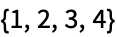Answer & check your solution
3.2Make a list of numbers up to 100. »
Expected output:
 Out[]=Answer & check your solution
3.3Use Range and Reverse to create {4, 3, 2, 1}»
Expected output:
 Out[]=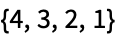Answer & check your solution
3.4Make a list of numbers from 1 to 50 in reverse order. »
Expected output:
 Out[]=Answer & check your solution
3.5Use Range, Reverse and Join to create {1, 2, 3, 4, 4, 3, 2, 1}»
Expected output:
 Out[]=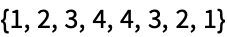Answer & check your solution
3.6Plot a list that counts up from 1 to 100, then down to 1. »
Expected output:
 Out[]=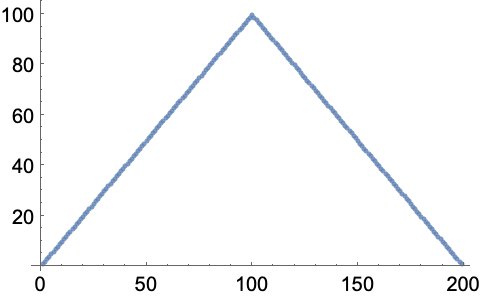Answer & check your solution
3.7Use Range and RandomInteger to make a list with a random length up to 10. »
Sample expected output:
 Out[]=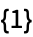Answer & check your solution
Expected output:
 Out[]=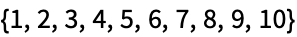Answer & check your solution
3.9Find a simpler form for Join[{1, 2}, Join[{3, 4}, {5}]]»
Expected output:
 Out[]=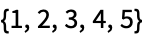Answer & check your solution
3.10Find a simpler form for Join[Range, Join[Range, Range]]»
Expected output:
 Out[]=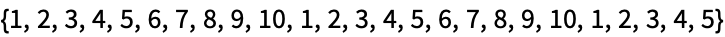Answer & check your solution
3.11Find a simpler form for Reverse[Join[Range, Reverse[Range]]]»
Expected output:
 Out[]=Answer & check your solution
+3.1Compute the reverse of the reverse of {1, 2, 3, 4}»
Expected output:
 Out[]=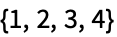Answer & check your solution
+3.2Use Range, Reverse and Join to create the list {1, 2, 3, 4, 5, 4, 3, 2, 1}»
Expected output:
 Out[]=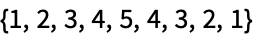Answer & check your solution
+3.3Use Range, Reverse and Join to create {3, 2, 1, 4, 3, 2, 1, 5, 4, 3, 2, 1}»
Expected output:
 Out[]=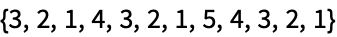Answer & check your solution
+3.4Plot the list of numbers {10, 11, 12, 13, 14}»
Expected output:
 Out[]=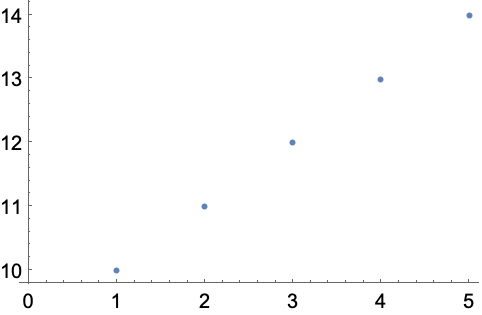Answer & check your solution
+3.5Find a simpler form for Join[Join[Range, Reverse[Range]], Range]»
Expected output:
 Out[]=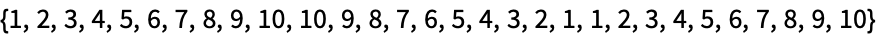Answer & check your solution
How does one read {1, 2, 3} out loud?
Usually “list 1 2 3”. “{” and “}” are called “braces” or “curly brackets”. “{” is “open brace” and “}” is “close brace”.
Is a list a function?
Yes. {1, 2, 3} is List[1, 2, 3]. But unlike, say, Plus, the function List doesn’t actually compute anything; it just comes back unchanged.
What is ListPlot plotting?
The values of successive list elements. The x value of each point gives the position in the list; the y value gives the value of that element.
How long can lists be?
As long as you want, until your computer runs out of memory.
• Range[m, n] generates numbers from m to n. Range[m, n, s] generates numbers from m to n in steps of s.
• Many computer languages have constructs like lists (often called “arrays”). But usually they only allow lists of explicit things, like numbers; you can’t have a list like {a, b, c} where you haven’t said what a, b and c are. You can in the Wolfram Language, though, because the Wolfram Language is symbolic.
• {a, b, c} is a list of elements in a definite order; {b, c, a} is a different list.
• Like in math, you can make theorems about Wolfram Language functions. For example, Reverse[Reverse[x]] is equal to x.